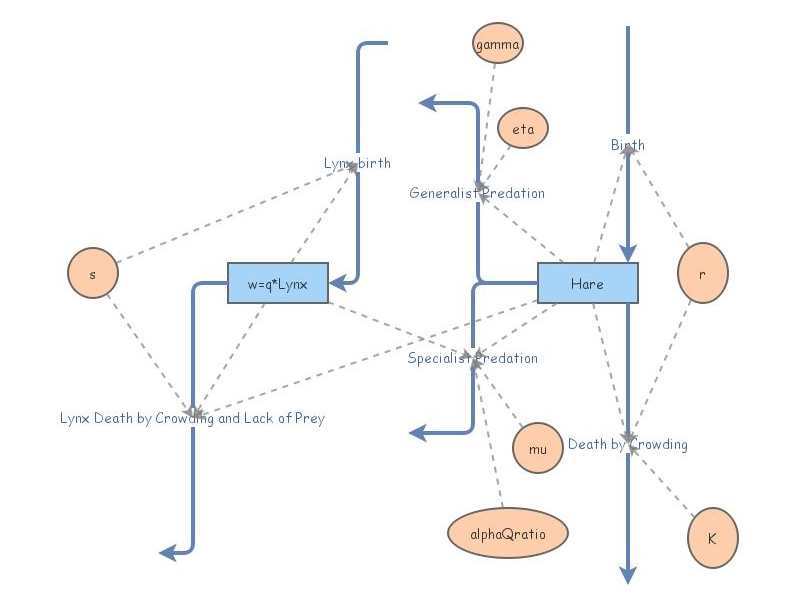Basic Model, Tyson Lynx and HareThe basic model of Modelling the Canada lynx and snowshoe hare population cycle: The role of specialist predators (Tyson, et al.) demonstrates logistic growth in prey, and in predator (with prey dependence for carrying capacity). But interestingly, one possibility is limit cycles, which mimic the cycling of the populations in nature.

The differential equations for the population of hare (x) is

x'(t) = rx(1-x/K)
- gamma x^2/(x^2+eta^2)
- alpha y x/(x+mu)

where K is the logistic carrying capacity of the prey (hare), in the absence of predation; the second term is a "generalist predation" term;  and the third term is the "specialist predation" (in the limit as the prey gets big, this becomes simply proportional to y (the lynx population)).

The differential equations for the population of lynx (y) is

y'(t) = sy(1- qy/x) = sy - sqy^2/x

for the predator (lynx), which is essentially logistic growth. Its growth term suggests exponential growth, but there is a loss term of the form sqy^2/x -- loss is proportional to population (crowding), and inversely proportional to prey density. As the hare population goes to zero, so shall the lynx....

As one can see, the prey density won't change if y=x/q. If the prey density were not changing at the same time, the system would be at equilibrium.

In this InsightMaker model, I scaled the second equation by multiplying by q, then replace y by w=qy throughout both equations. This requires a slight change in the prey equation -- alpha replaced by the ratio of alpha/q.  (I used my favorite mathematical trick, of multiplying by the appropriate form of 1!)

So what we're really looking at here is the system

x'(t) = rx(1-x/K)
- gamma x^2/(x^2+eta^2)
- alpha/q w x/(x+mu)
w'(t) = sw(1- w/x)

where w(t)=qy(t).

Tyson, et al. took q to be about 212 for hare and lynx -- so that it requires about 212 hare to allow for one lynx to survive at "equilibrium".

However, when alpha -- the hares/lynx/year -- gets sufficiently large (e.g. 1867 -- and that does seem like a lot of hares per lynx per year...:), limit cycles develop (rather than a stable equilibrium). This means that the populations oscillate about the equilibrium values, rather than stabilize at those values.

Author: Andy Long, Northern Kentucky University (2020)

Reference: Tyson, Rebecca, Sheena Haines,  Karen Hodges. Modelling the Canada lynx and snowshoe hare population cycle: The role of specialist predators. Theoretical Ecology. 3, 97–111 (2010). https://doi.org/10.1007/s12080-009-0057-1

Resource: A comparable Mathematica model can be found at  http://ceadserv1.nku.edu/longa//classes/mat375/days/Mathematica/BasicModel.nb,
which allows one to experiment a little more easily than one can with this InsightMaker model.